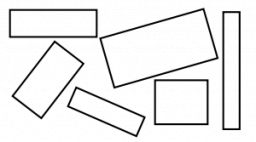# Peter's rectangle

Peter had a rectangle 2 cm wide and of unknown length. The line had a 2 cm rectangle whose length was equal to the perimeter of Peter's rectangle. When they put the rectangles together with their widths, they got a new rectangle with a circumference of 63 cm. Find the area of Peter's rectangle.

S1 =  17 cm2

### Step-by-step explanation:Did you find an error or inaccuracy? Feel free to write us. Thank you!

Tips for related online calculators
Do you have a linear equation or system of equations and looking for its solution? Or do you have a quadratic equation?

#### Grade of the word problem:

We encourage you to watch this tutorial video on this math problem: